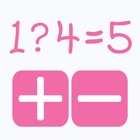Quick Math - The free and simple super casual mathematical equation game

• SEARCH TYPE

All iPhone applications categories

All iPhone games categories# Quick Math - The free and simple super casual mathematical equation game

0 0

## Screenshots

Description

How fast could you solve simple mathematical equation?

Quick Math is a simple and straightforward game, you just need to select the operator to complete the shown equation.

Enjoy the game. :)